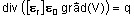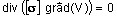# Formulation: general aspects

## Introduction

This paragraph introduces the concepts of state variable, formulation and model of formulation.

## Concept of state variable

From a theoretical point of view, each physical application has a system of equations.

To solve this system of equations variables of calculation, named state variables, are used. They reduce the system of equations to one or two equations. (It will then be possible to compute all electromagnetic quantities starting from these variables).

Examples:

• electric application: the state variable is the electric potential V
• thermal application: the state variable is the temperature T

## Concept of formulation

There is a formulation for each region in Flux that shows:

• what is the state variable?
• what equation is solved and how?

Examples:

• electrostatic application: (formulation ES3SCA)
• the state variable is the electric potential V (written PHI1 in Flux 3D)
• the solved equation is the following equation:• electric conduction application: (formulation EC3SCA)
• the state variable is the electric potential V (written PHI1 in Flux 3D)
• the solved equation is the following equation:## Formulation and applications

Only one method of solving is provided for electrical applications and thermal applications. That results in only one Flux formulation for all regions of the domain (see previous examples).

Various methods of solving are provided for magnetic applications. This results in the choice of a Flux formulation for each region of the domain (see following block).

## Concept of model

Various formulations provided in magnetic applications can be gathered and we then speak about a model of formulation .

Two models provided within magnetic applications are:

• the vector model that uses: the magnetic vector potential A and the electric scalar potential V

• the scalar model that uses: various magnetic scalar potentials ϕ and the electric vector potential T